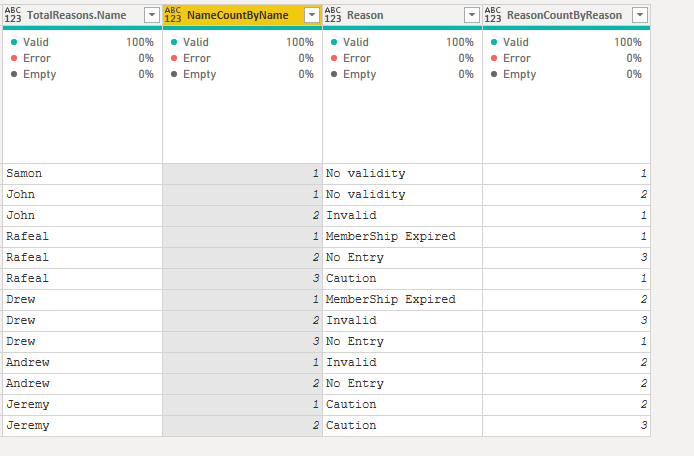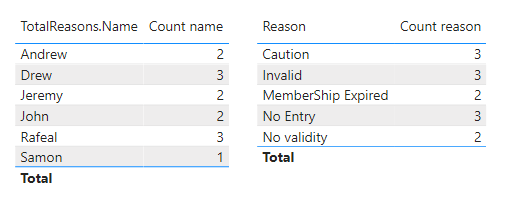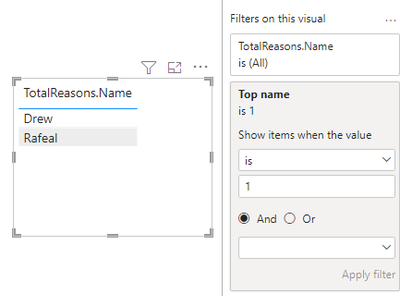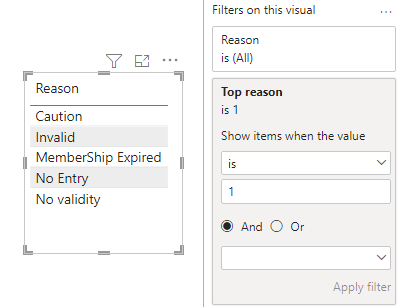cancel
Showing results for
Did you mean:Anonymous
Not applicable

## Top N from the columnIssue I haveHi Experts,

Test Data file: PowerBi.Pbix

I have data where the all reasons are in one column and I need to find the TOP 10 along with other columns where I need top 10, SD, mean etc, Reason count by Reason ( is the index created grouped by Reason).

I tried following adapted from the solution in the Community Forum.

1. I need help to get TOP 5 reasons in the column and

2. When I do not have to create the index in the query too, if possible so other measures can be done without adding the steps of Index and group by.

``````RankReason =
IF (
ISINSCOPE ( 'Data'[ReasonCountByReason]),
RANKX (
CALCULATETABLE (
VALUES ( Data[ReasonCountByReason] ),
ALLSELECTED ( Data[ReasonCountByReason] )
),
[ReasonCountByReason]
),
IF (
ISINSCOPE ( 'Data'[ReasonCountByReason] ),
VAR TotalReasons = Data[TopReasons]
RETURN
CALCULATE (
RANKX (
VALUES ( 'Data'[ReasonCountByReason] ),
[TopReasons],
Data[TopReasons]
),
ALLSELECTED ()
)
)
)

-- Did not work``````1 ACCEPTED SOLUTIONCommunity Support

Hi, @Anonymous

Please try the following methods. You can solve it directly with Dax without creating indexes in the power query.

Measure:

``````Count name =
CALCULATE (
COUNT ( Data[TotalReasons.Name] ),
FILTER (
ALL ( Data ),
[TotalReasons.Name] = SELECTEDVALUE ( Data[TotalReasons.Name] )
)
)
``````
``````Count reason =
CALCULATE (
COUNT ( Data[Reason] ),
FILTER ( ALL ( Data ), [Reason] = SELECTEDVALUE ( Data[Reason] ) )
)
````````Rank name = RANKX(ALLSELECTED(Data[TotalReasons.Name]),[Count name],,DESC)``
``Top name = IF([Rank name]<=2,1,0)``

Put the measure of Top name into the name's view and set it equal to 1.Is this the result you want for Top 2 name?

``Rank reason = RANKX(ALLSELECTED(Data[Reason]),[Count reason],,DESC)``
``Top reason = IF([Rank reason]<=5,1,0)``Is this the result you want for Top 5 reason?

Best Regards,

Community Support Team _Charlotte

If this post helps, then please consider Accept it as the solution to help the other members find it more quickly.

2 REPLIES 2Anonymous
Not applicable
Spoiler
@v-zhangti  Thanks a lot. Much appreciated.Community Support

Hi, @Anonymous

Please try the following methods. You can solve it directly with Dax without creating indexes in the power query.

Measure:

``````Count name =
CALCULATE (
COUNT ( Data[TotalReasons.Name] ),
FILTER (
ALL ( Data ),
[TotalReasons.Name] = SELECTEDVALUE ( Data[TotalReasons.Name] )
)
)
``````
``````Count reason =
CALCULATE (
COUNT ( Data[Reason] ),
FILTER ( ALL ( Data ), [Reason] = SELECTEDVALUE ( Data[Reason] ) )
)
````````Rank name = RANKX(ALLSELECTED(Data[TotalReasons.Name]),[Count name],,DESC)``
``Top name = IF([Rank name]<=2,1,0)``

Put the measure of Top name into the name's view and set it equal to 1.Is this the result you want for Top 2 name?

``Rank reason = RANKX(ALLSELECTED(Data[Reason]),[Count reason],,DESC)``
``Top reason = IF([Rank reason]<=5,1,0)``Is this the result you want for Top 5 reason?

Best Regards,

Community Support Team _Charlotte

If this post helps, then please consider Accept it as the solution to help the other members find it more quickly.